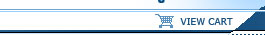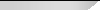NOTICE: TITANCE.COM WILL BE DOWN FOR SCHEDULED MAINTENANCE BEGINNING AT 6:00PM ET ON WEDNESDAY, JULY 24TH.Online CoursesContractors/TradesArchitectsEngineersHome Study Course BooksContractors/TradesArchitectsEngineersLive WebinarsArchitectsEngineersLive SeminarsContractors/TradesArchitectsEngineers
 If you have any questions please call a Titan Representative 1-800-960-8858

Pipe Flow Calculations Part II - Partially Full Pipe Flow Calculations (3 credit hours)
The Manning equation is used for a variety of types of uniform open channel flow, including gravity flow in pipes, the topic of this course.  This 3 hour course includes a review of the Manning equation, along with presentation of equations for calculating the cross-sectional area, wetted perimeter, and hydraulic radius for flow of a specified depth in a pipe of known diameter. Numerous worked examples illustrate the use of these equations together with the Manning equation to calculate flow rate and velocity, normal depth, minimum required pipe diameter, required pipe slope or full flow Manning roughness coefficient for partially full pipe flow.

This course is intended for hydrologists, civil engineers, hydraulic engineers, highway engineers, environmental engineers and mechanical engineers.  After completing this course you will have knowledge about the equations for calculating area, wetted perimeter, and hydraulic radius for partially full pipe flow and equations for calculating the Manning roughness coefficient at a given depth to diameter ratio, with a known value of the Manning roughness coefficient for full pipe flow.  Practice in the use of the Manning equation for a variety of partially full pipe flow calculations will be gained through several worked examples.
As prepared for Titan Coursework this series has been separated into separate courses and may be taken out of sequence, each course independent from another.
 Harlan Bengtson
At the conclusion of this course, the student will:

• Be able to calculate the cross-sectional area of flow, wetted perimeter, and hydraulic radius for less than half full flow at a given depth in a pipe of given diameter.
• Be able to calculate the cross-sectional area of flow, wetted perimeter, and hydraulic radius for more than half full flow at a given depth in a pipe of given diameter.
• Be able to use Figure 6 in the course material to determine the flow rate at a given depth of flow in a pipe of known diameter if the full pipe flow rate is known or can be calculated.
• Be able to use Figure 6 in the course material to determine the average water velocity at a given depth of flow in a pipe of known diameter if the full pipe average velocity is known or can be calculated.
• Be able to calculate the Manning roughness coefficient for a given depth of flow in a pipe of known diameter, with a known Manning roughness coefficient for full pipe flow.
• Be able to use the Manning equation to calculate the flow rate and average velocity for flow at a specified depth in a pipe of specified diameter, with known pipe slope and full pipe Manning roughness coefficient.
• Be able to calculate the normal depth for a specified flow rate of water through a pipe of known diameter, slope, and full pipe Manning roughness coefficient.
• Be able to calculate the minimum required pipe diameter for a specified flow rate of water through a pipe of known slope, full pipe Manning roughness coefficient and a target value for y/D.
• Be able to calculate the required pipe slope for a specified flow rate of water through a pipe of known diameter, depth of flow, and full pipe Manning roughness coefficient.
• Be able to calculate the value of the full pipe Manning roughness coefficient for a specified flow rate of water through a pipe of known diameter, slope, and depth of flow.
• Be able to carry out the calculations in the above learning objectives using either U.S. units or S.I. units
As prepared for Titan Coursework this series has been separated into separate courses and may be taken out of sequence, each course independent from another.Titan Continuing Education, Inc. | 1519 Dale Mabry Hwy, Ste 201 Lutz, FL 33548 | Toll Free: 800.960.8858 | Email: info@TitanCE.com.